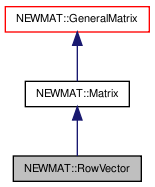# NEWMAT::RowVector Class Reference

`#include <newmat.h>`

Inheritance diagram for NEWMAT::RowVector:[legend]

## Detailed Description

Definition at line 847 of file newmat.h.

List of all members.

## Public Member Functions

RowVector ()
~RowVector ()
RowVector (ArrayLengthSpecifier n)
RowVector (const BaseMatrix &)
RowVector (const RowVector &gm)
void operator= (const BaseMatrix &)
RowVectoroperator= (Real f)
RowVectoroperator= (const RowVector &m)
Real & operator() (int)
Real & element (int)
Real operator() (int) const
Real element (int) const
MatrixType Type () const
void GetCol (MatrixRowCol &)
void GetCol (MatrixColX &)
void NextCol (MatrixRowCol &)
void NextCol (MatrixColX &)
void RestoreCol (MatrixRowCol &)
void RestoreCol (MatrixColX &c)
GeneralMatrixTranspose (TransposedMatrix *, MatrixType)
void ReSize (int)
void ReSize (int, int)
void ReSize (const GeneralMatrix &A)
Real * nric () const
void CleanUp ()
void MiniCleanUp ()
void operator+= (const Matrix &M)
void operator-= (const Matrix &M)
void operator+= (Real f)
void operator-= (Real f)
void swap (RowVector &gm)

## Private Member Functions

GeneralMatrixImage () const

## Constructor & Destructor Documentation

 NEWMAT::RowVector::RowVector ( )

Definition at line 851 of file newmat.h.

Referenced by Image().

 NEWMAT::RowVector::~RowVector ( )

Definition at line 852 of file newmat.h.

 NEWMAT::RowVector::RowVector ( ArrayLengthSpecifier n )

Definition at line 853 of file newmat.h.

 NEWMAT::RowVector::RowVector ( const BaseMatrix & M )

Definition at line 101 of file newmat4.cpp.

 NEWMAT::RowVector::RowVector ( const RowVector & gm )

Definition at line 855 of file newmat.h.

## Member Function Documentation

 void NEWMAT::RowVector::CleanUp ( ) ` [virtual]`

Reimplemented from NEWMAT::GeneralMatrix.

Definition at line 905 of file newmat4.cpp.

 Real NEWMAT::RowVector::element ( int n ) const

Definition at line 776 of file newmat6.cpp.

 Real & NEWMAT::RowVector::element ( int n )

Definition at line 769 of file newmat6.cpp.

Referenced by NEWMAT::SVD().

 void NEWMAT::RowVector::GetCol ( MatrixColX & mrc ) ` [virtual]`

Reimplemented from NEWMAT::Matrix.

Definition at line 538 of file newmat3.cpp.

 void NEWMAT::RowVector::GetCol ( MatrixRowCol & mrc ) ` [virtual]`

Reimplemented from NEWMAT::Matrix.

Definition at line 526 of file newmat3.cpp.

 GeneralMatrix * NEWMAT::RowVector::Image ( ) const` [private, virtual]`

Reimplemented from NEWMAT::Matrix.

Definition at line 795 of file newmat4.cpp.

 void NEWMAT::RowVector::MiniCleanUp ( ) ` [virtual]`

Reimplemented from NEWMAT::GeneralMatrix.

Definition at line 884 of file newmat.h.

 void NEWMAT::RowVector::NextCol ( MatrixColX & mrc ) ` [virtual]`

Reimplemented from NEWMAT::Matrix.

Definition at line 550 of file newmat3.cpp.

 void NEWMAT::RowVector::NextCol ( MatrixRowCol & mrc ) ` [virtual]`

Reimplemented from NEWMAT::Matrix.

Definition at line 547 of file newmat3.cpp.

 Real* NEWMAT::RowVector::nric ( ) const

Definition at line 881 of file newmat.h.

 Real NEWMAT::RowVector::operator() ( int n ) const

Definition at line 197 of file newmat6.cpp.

 Real & NEWMAT::RowVector::operator() ( int n )

Definition at line 85 of file newmat6.cpp.

 void NEWMAT::RowVector::operator+= ( Real f )

Reimplemented from NEWMAT::Matrix.

Definition at line 889 of file newmat.h.

 void NEWMAT::RowVector::operator+= ( const Matrix & M )

Reimplemented from NEWMAT::Matrix.

Definition at line 887 of file newmat.h.

 void NEWMAT::RowVector::operator-= ( Real f )

Reimplemented from NEWMAT::Matrix.

Definition at line 890 of file newmat.h.

 void NEWMAT::RowVector::operator-= ( const Matrix & M )

Reimplemented from NEWMAT::Matrix.

Definition at line 888 of file newmat.h.

 RowVector& NEWMAT::RowVector::operator= ( const RowVector & m )

Definition at line 858 of file newmat.h.

 RowVector& NEWMAT::RowVector::operator= ( Real f )

Reimplemented from NEWMAT::Matrix.

Definition at line 857 of file newmat.h.

 void NEWMAT::RowVector::operator= ( const BaseMatrix & X )

Reimplemented from NEWMAT::Matrix.

Definition at line 369 of file newmat6.cpp.

Referenced by operator=().

 void NEWMAT::RowVector::ReSize ( const GeneralMatrix & A ) ` [virtual]`

Reimplemented from NEWMAT::Matrix.

Definition at line 317 of file newmat4.cpp.

 void NEWMAT::RowVector::ReSize ( int nr, int nc ) ` [virtual]`

Reimplemented from NEWMAT::Matrix.

Definition at line 278 of file newmat4.cpp.

 void NEWMAT::RowVector::ReSize ( int nc )

Definition at line 272 of file newmat4.cpp.

Referenced by ReSize().

 void NEWMAT::RowVector::RestoreCol ( MatrixColX & c ) ` [virtual]`

Reimplemented from NEWMAT::Matrix.

Definition at line 562 of file newmat3.cpp.

 void NEWMAT::RowVector::RestoreCol ( MatrixRowCol & ) ` [virtual]`

Reimplemented from NEWMAT::Matrix.

Definition at line 875 of file newmat.h.

 void NEWMAT::RowVector::swap ( RowVector & gm )

Definition at line 891 of file newmat.h.

Referenced by NEWMAT::swap().

 GeneralMatrix * NEWMAT::RowVector::Transpose ( TransposedMatrix * , MatrixType mt ) ` [virtual]`

Reimplemented from NEWMAT::GeneralMatrix.

Definition at line 68 of file newmat5.cpp.

 MatrixType NEWMAT::RowVector::Type ( ) const` [virtual]`

Reimplemented from NEWMAT::Matrix.

Definition at line 432 of file newmat4.cpp.

The documentation for this class was generated from the following files:

 newmat11b Generated Mon May 9 04:54:19 2016 by Doxygen 1.6.3